0

# 猿创征文|OpenCV编程&mdash;&mdash;计算机视觉的登堂入室

``````OpenCV（Open Source Computer Vision）
``````

## 一、接触图像

• opencv_core模块：包含核心功能，尤其是底层数据结构和算法函数。
• opencv_imgproc模块：包含图像处理函数。
• opencv_highgui模块：包含读写图像及视频的函数，以及操作图形用户界面函数。
• opencv_features2d模块：包含兴趣点检测子、描述子以及兴趣点匹配框架。

``````cv::Mat image = cv::imread("/home/dzh/QtOpenCV/image.png",1);
cv::namedWindow("Image");
cv::imshow("Image", image);
cv::waitKey(0);
``````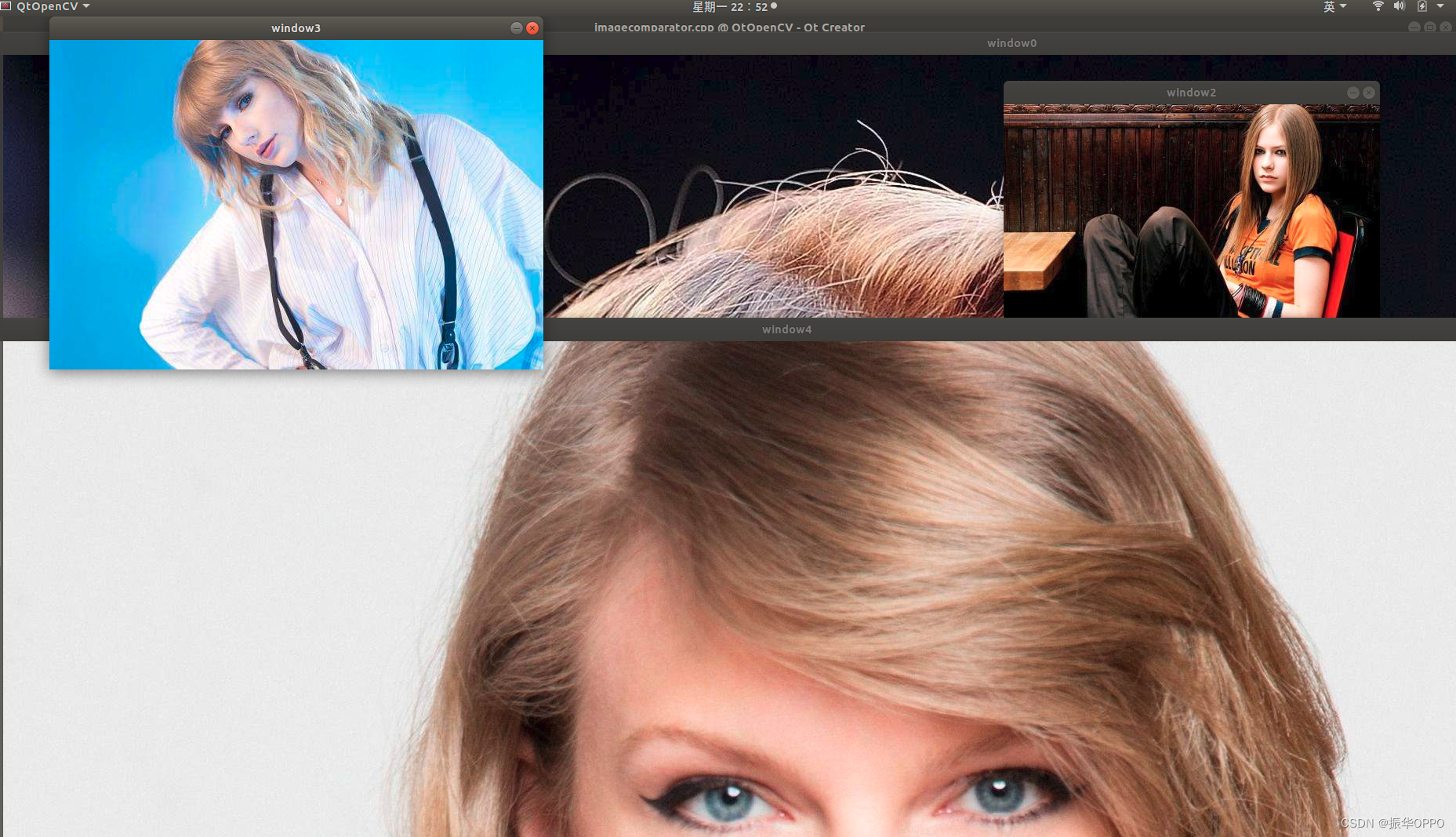## 二、操作像素

``````// 颜色缩减[指针遍历]voidMainWindow::colorReduce(cv::Mat &image, cv::Mat &result,int div){// 行数int nl = image.rows;// 每行的元素个数（像素个数 x 通道数）int nc = image.cols * image.channels();// 左移位数int n =0;// 遍历每一行for(int j =0; j < nl; j++){// 得到第j行的首地址
uchar* data = image.ptr<uchar>(j);// 求解pow的反函数for(int k =1; k <=8; k++){if(pow(2, k)== div){
n = k;break;}elseif(pow(2, k)> div){
n = k -1;break;}}// 掩码左移
uchar mask =0xFF<< n;for(int i =0; i < nc; i++){//            data[i] = data[i] / div * div + div / 2;
data[i]=(data[i]& mask)+ div /2;}}}// 图像运算voidMainWindow::imageProc(cv::Mat &image1, cv::Mat &image2, cv::Mat &result){
result = image1 *0.8+ image2 *0.2;}// 感兴趣区域voidMainWindow::imageROI(cv::Mat &image1, cv::Mat &image2){// 相当于截取图像的一个区域
cv::Mat roi =image1(cv::Rect(280,260, image2.cols, image2.rows));
``````

## 三、基于类的图像处理

``````voidHistogram1D::process(){
cout <<"图像不存在"<< endl;return;}else{
cout <<"图像的宽度："<< image.cols <<"图像的高度："<< image.rows <<"像素总数："<< image.cols * image.rows << endl;}
Histogram1D hc;
cv::Mat imageROI;// 获取感兴趣区域
imageROI =image(cv::Rect(160,300,100,120));int minSat =65;// 彩色直方图
cv::MatND colorhist = hc.getHueHistogram(imageROI, minSat);
ContentFinder finder;
finder.setHistogram(colorhist);
cv::imshow("Detect", detect);
cv::Mat hsv;
cv::cvtColor(image, hsv, CV_BGR2HSV);
vector<cv::Mat> v;
cv::split(hsv, v);
cv::Mat result;
cv::threshold(v, v, minSat,255, cv::THRESH_BINARY);
result = finder.find(hsv,0.0f,180.0f, channels,1);
cv::bitwise_and(result, v, result);
cv::Rect rect(110,260,35,40);
cv::rectangle(image, rect, cv::Scalar(255,255,255));
cv::TermCriteria criteria(cv::TermCriteria::MAX_ITER,10,0.01);
cv::meanShift(result, rect, criteria);//    cv::imshow("origin", colorhist);
cv::imshow("output", result);
cv::waitKey(0);}
``````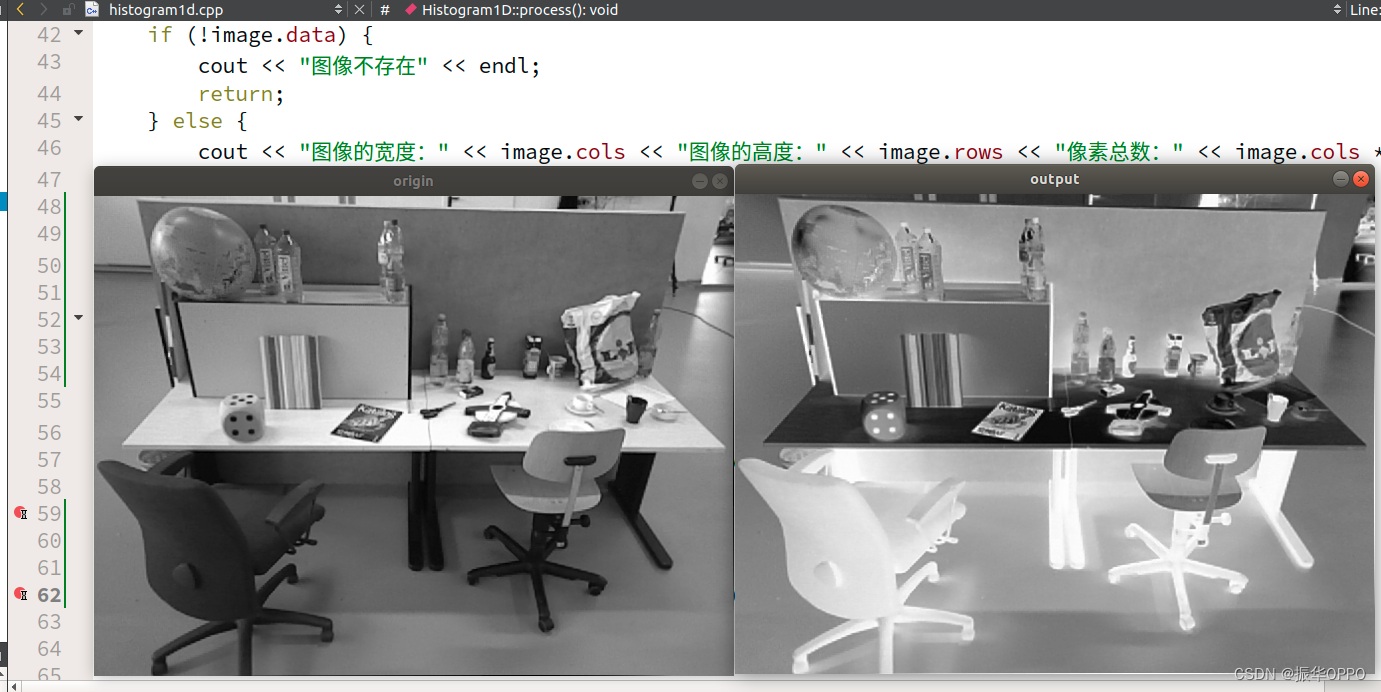## 四、使用直方图统计像素

``````cv::Mat Histogram3D::getHistogramImage(cv::Mat &image){// 先求取直方图(256x256x256)
cv::MatND hist =getHistogram(image);// 设置最大值和最小值double maxVal = INT_MIN;double minVal = INT_MAX;// 创建显示图像256x256x3
cv::Mat histImg(histSize, histSize, CV_8UC3, cv::Scalar(255,255,255));int hpt =static_cast<int>(0.9* histSize);// 3D->2D，可以减少一个维度
vector<vector<float>>vec(3,vector<float>(256));// b通道for(int i =0; i <256; i++){// g通道for(int j =0; j <256; j++){// r通道for(int k =0; k <256; k++){
vec[i]+= hist.at<float>(i, j, k);
vec[j]+= hist.at<float>(i, j, k);
vec[k]+= hist.at<float>(i, j, k);}}}// 先遍历每一行for(int i =0; i < vec.size(); i++){// 对于每一行直方图取最大值和最小值
cv::minMaxLoc(vec[i],&minVal,&maxVal,0,0);
cout <<"maxVal:"<< maxVal <<",minVal:"<< minVal << endl;// 再遍历每一列for(int j =0; j < vec[i].size(); j++){float val = vec[i][j];int intensity =static_cast<int>(val * hpt / maxVal);
cv::Scalar color;
color[i]=255;
cv::line(histImg, cv::Point(j, histSize[i]), cv::Point(j, histSize[i]- intensity), color);}}return histImg;}
``````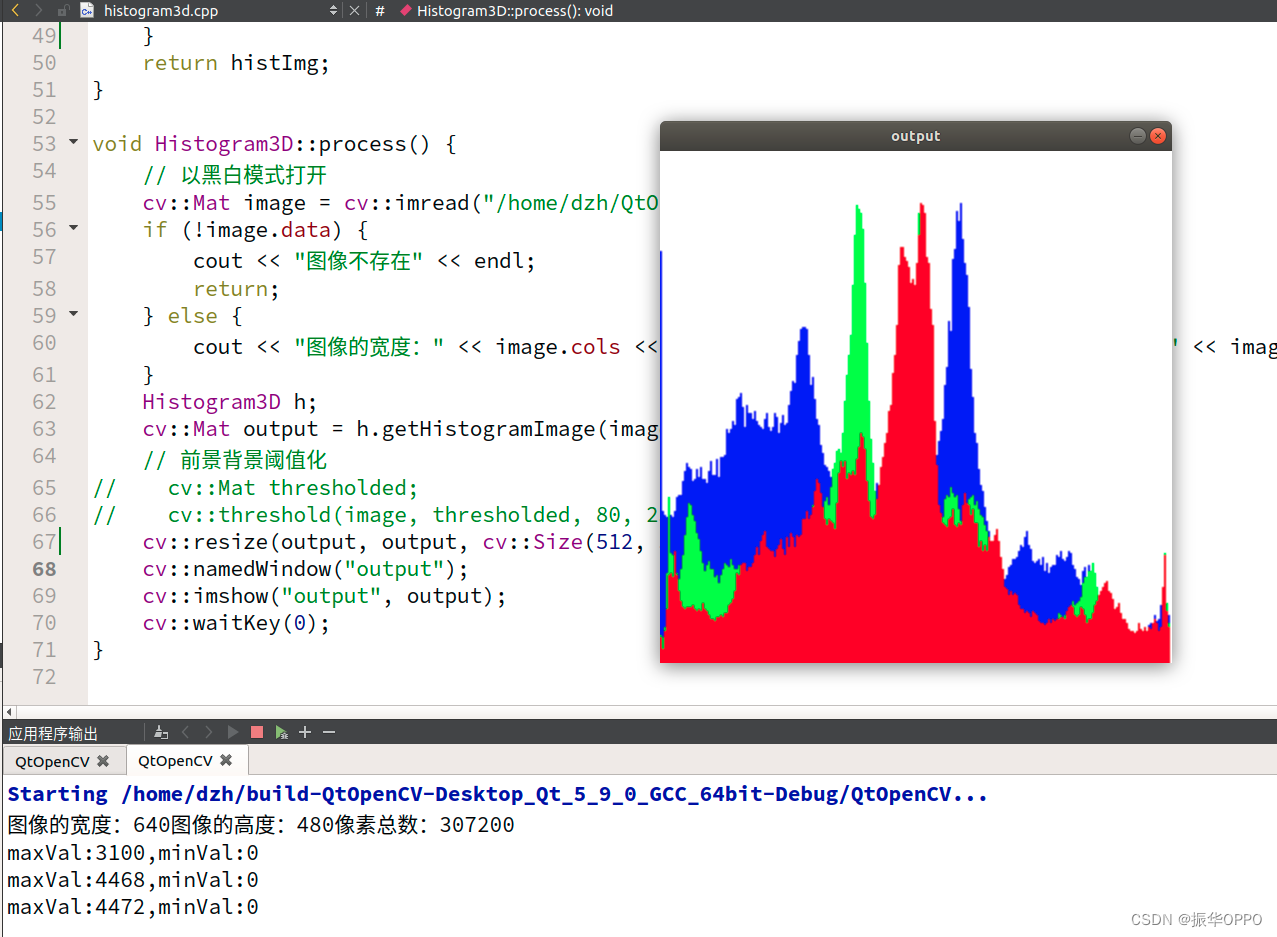## 五、基于形态学运算的图像变换

``````cv::Mat MorphoFeatures::getEdges(cv::Mat &image){// 得到梯度图
cv::Mat result;
cv::morphologyEx(image, result, cv::MORPH_GRADIENT, cv::Mat());// 阈值化得到二值图像applyThreshold(result);return result;}voidMorphoFeatures::setThreshold(int value){
threshold = value;}

cv::Mat MorphoFeatures::getCorners(cv::Mat &image){
cv::Mat result;
cv::dilate(image, result, cross);
cv::erode(result, result, diamond);

cv::Mat result2;
cv::dilate(image, result2, x);
cv::erode(result2, result, square);

cv::absdiff(result2, result, result);applyThreshold(result);return result;}
``````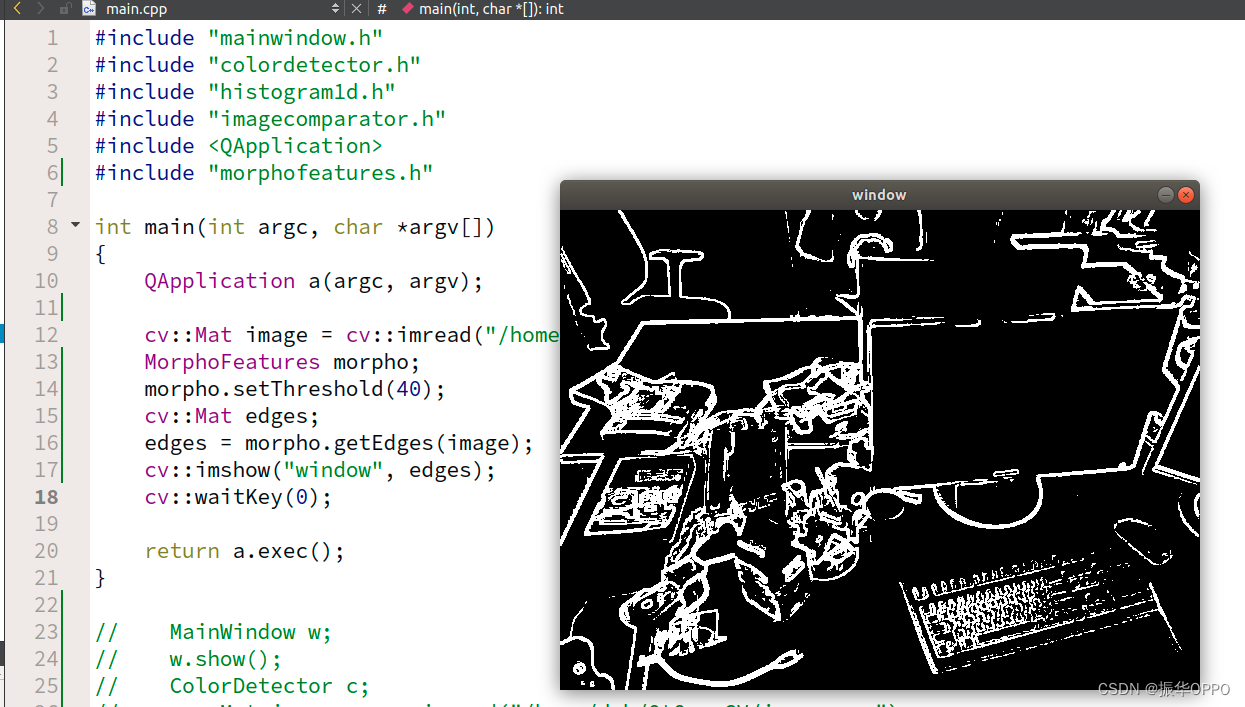## 六、图像滤波

``````cv::Mat contoursInv;
cv::threshold(contours, contoursInv,128,255, cv::THRESH_BINARY_INV);
cv::imshow("Inv", contoursInv);
cv::Mat result1, result2;
cv::Sobel(image, result1, CV_8U,1,0,3,0.4,128);
cv::Sobel(image, result2, CV_8U,0,1,3,0.4,128);
cv::imshow("result1", result1);
cv::imshow("result2", result2);
cv::Mat sobelX, sobelY;
cv::Sobel(image, sobelX, CV_8U,1,0);
cv::Sobel(image, sobelY, CV_8U,0,1);
cv::Mat sobel;
sobel = sobelX + sobelY;
cv::imshow("Sobel", sobel);
``````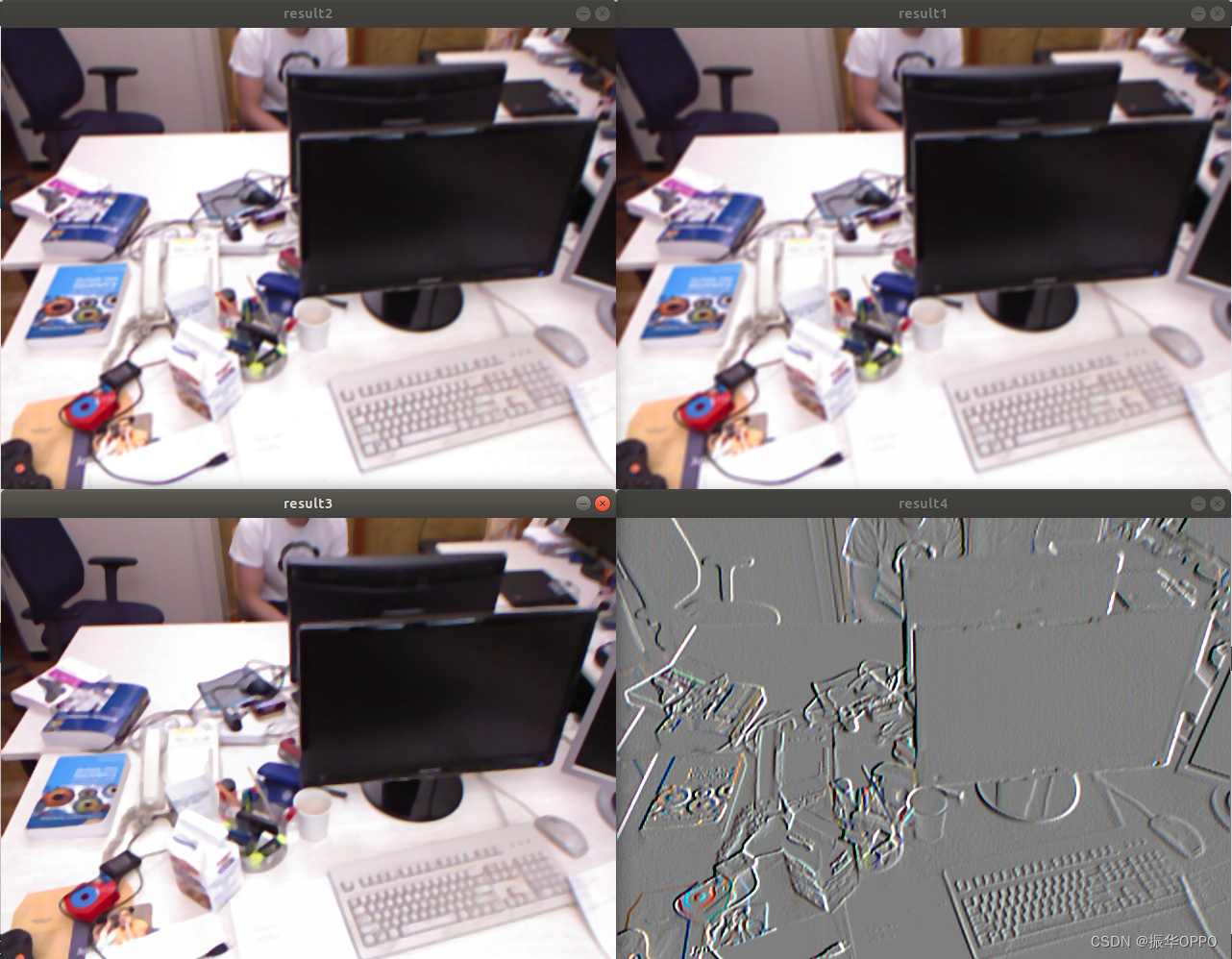## 七、检测并匹配特征点

``````// 计算Harris角点voidHarrisDetector::detect(cv::Mat &image){
cv::cornerHarris(image, cornerStrength, neighbourhood, aperture, k);double minStrength;
cv::minMaxLoc(cornerStrength,&minStrength,&maxStregth);
cv::Mat dilated;
cv::dilate(cornerStrength, dilated, cv::Mat());
cv::compare(cornerStrength, dilated, localMax, cv::CMP_EQ);}// 由Harris值获取角点图
cv::Mat HarrisDetector::getCornerMap(double qualityLevel){
cv::Mat cornerMap;
threshold = qualityLevel * maxStregth;
cv::threshold(cornerStrength, cornerTh, threshold,255, cv::THRESH_BINARY);
cornerTh.convertTo(cornerMap, CV_8U);
cv::bitwise_and(cornerMap, localMax, cornerMap);return cornerMap;}
``````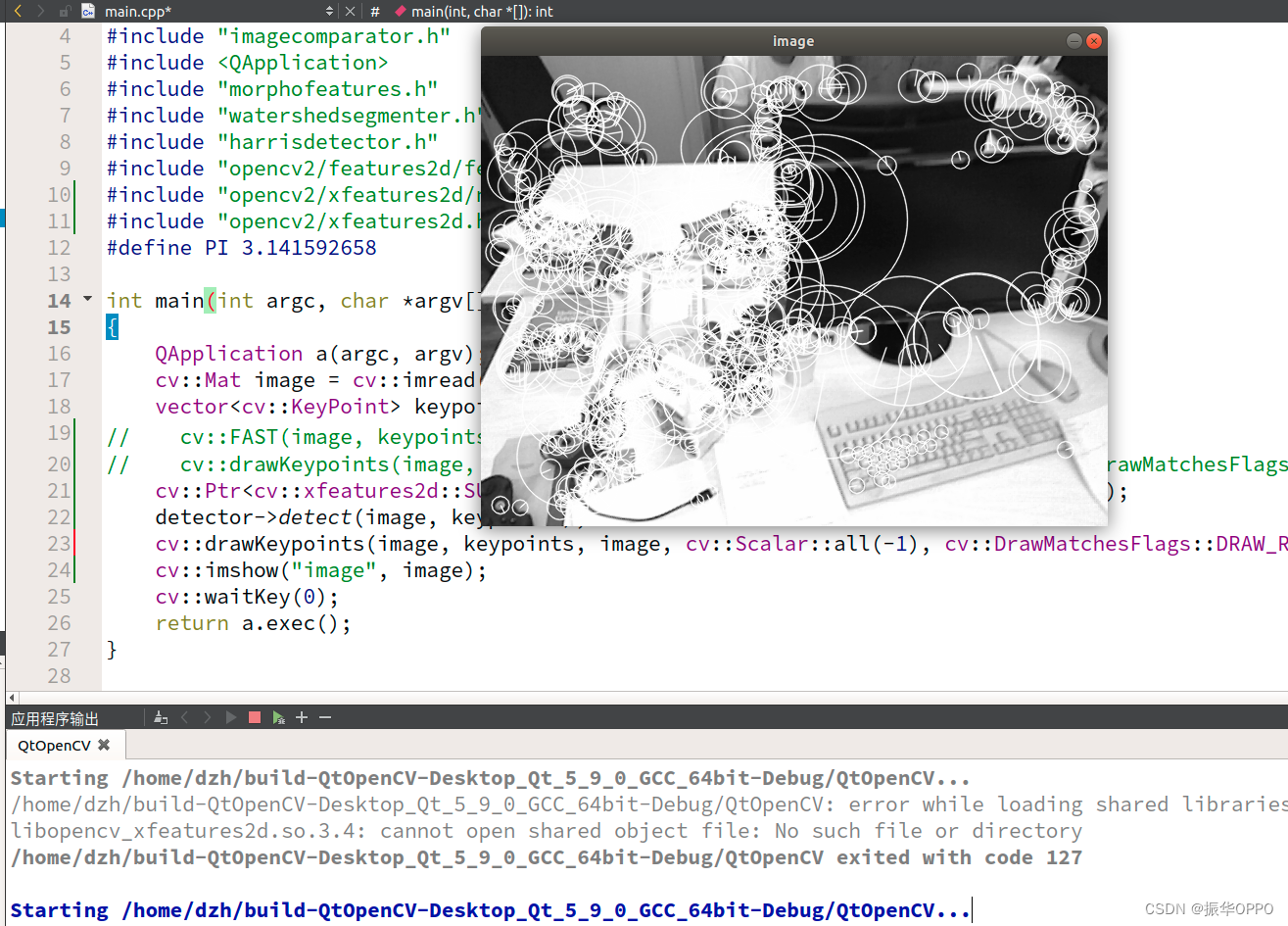### “猿创征文|OpenCV编程&mdash;&mdash;计算机视觉的登堂入室”的评论:

##### 关于作者##### overfit同步小助手